# AP Physics C: Mechanics Practice Test 1

### Test Information10 questions13 minutes

1. A rock is dropped off a cliff and falls the first half of the distance to the ground in t1 seconds. If it falls the second half of the distance in t2 seconds, what is the value of t2/t1? (Ignore air resistance.)

2. A box of mass m slides on a horizontal surface with initial speed v0. It feels no forces other than gravity and the force from the surface. If the coefficient of kinetic friction between the box and the surface is μ, how far does the box slide before coming to rest?

3. An object initially at rest experiences a time-varying acceleration given by a = (2 m/s3)t for t ≥ 0. How far does the object travel in the first 3 seconds?

4. Which of the following conditions will ensure that angular momentum is conserved?

I. Conservation of linear momentum

II. Zero net external force

III. Zero net external torque

5.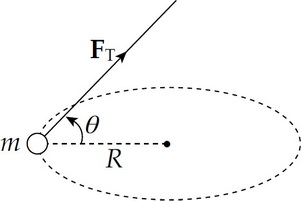In the figure shown, a tension force FT causes a particle of mass m to move with constant angular speed ω in a circular path (perpendicular to the page) of radius R. Which of the following expressions gives the magnitude of FT?

6. An object (mass = m) above the surface of the Moon (mass = M) is dropped from an altitude h equal to the Moon's radius (R). With what speed will the object strike the lunar surface?

7.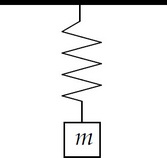The figure above shows a linear spring anchored to the ceiling. If the mass of the block hanging from its lower end is doubled, what effect will this change have on the block's equilibrium position and oscillation period?

8.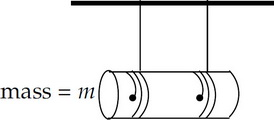A uniform cylinder of mass m and radius r unrolls without slipping from two strings tied to a vertical support. If the rotational inertia of the cylinder is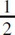mr2, find the acceleration of its center of mass.

9.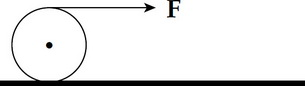A uniform cylinder, initially at rest on a frictionless, horizontal surface, is pulled by a constant force F from time t = 0 to time t = T. From time t = T on, this force is removed. Which of the following graphs best illustrates the speed, v, of the cylinder's center of mass from t = 0 to t = 2T?

10. An engine provides 10 kW of power to lift a heavy load at constant velocity a distance of 20 m in 5 s. What is the mass of the object being lifted?